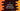# C programming structure explanation with example### Structure in C, explanation with example :

The structure is a user-defined data type in C. For example, suppose we want to store numbers from 1 to 100. We will use one integer array of size 100. But if we need to store the details of 100 students, each detail will include name,_ age, and _marks for a specific subject, what we will do? To solve such problems, we can use Structure.

### Creating a simple structure :

``````struct Student{
char name;
int age;
int marks;
};``````

It will create one structure Student. It will create one user-defined data type, but it will not allocate any memory. For that, we need to create variables of this data type.

### Declaring variables :

We can declare variables of type Student as below :

``````#include<stdio.h>

struct Student{
char name;
int age;
int marks;
};

int main(){
struct Student class10;
struct Student alex,bryan,chandler;
}``````
• In the above example, we have created one array class10 of type Student and size 10. Means, it has 10 elements and each element is of type Student.
• We have also created few other Student variables_ alex, bryan, and chandler_.

We can also declare all variables while defining the structure type like below :

``````#include<stdio.h>

struct Student{
char name;
int age;
int marks;
}class10,alex,bryan,chandler;

int main(){

}``````

### Using ‘typedef’ in structure :

In the above example, we use the struct keyword while declaring a variable like :

``````struct Student class10;
struct Student alex,bryan,chandler;``````

We can use typedef keyword to omit the repeated use of struct keyword like below :

``````typedef struct {
char name;
int age;
int marks;
}Student;

int main(){
Student class10;
Student alex,bryan,chandler;
}``````

The code looks much cleaner now. Isn’t it?

### Accessing values of a structure variable :

We can use one dot (’.‘) to access all items lies inside a structure variable. For example, if we want to access the name of a Student ‘alex’, we can use alex.name. Similarly, to set a value we can use same a dot in the same way. Let’s try it with an example :

``````#include<stdio.h>
#include<string.h>

typedef struct {
char name;
int age;
int marks;
}Student;

int main(){
Student chandler;

strcpy(chandler.name,"Chandler Bing");
chandler.age = 20;
chandler.marks = 87;

printf("Name : %s\n",chandler.name);
printf("Age : %d\n",chandler.age);
printf("Marks : %d\n",chandler.marks);
}``````

It will print the below output :

``````Name : Chandler Bing
Age : 20
Marks : 87``````

So, basically, we are assigning values to the variable and then retrieving them again.

### Passing structure variable to a function :

We can pass a structure variable to a different function in the same way we pass other variables to a function. Let’s explore this with an example :

``````#include<stdio.h>
#include<string.h>

typedef struct {
char name;
int age;
int marks;
}Student;

void printAllName(Student students[],int count){
int i;

for(i=0; i<count; i++){
printf("Name : %s , Age : %d , Marks : %d\n",students[i].name,students[i].age,students[i].marks);
}
}

int main(){
Student class10;

strcpy(class10.name,"Chandler");
class10.age = 20;
class10.marks = 87;

strcpy(class10.name,"Edward");
class10.age = 22;
class10.marks = 77;

strcpy(class10.name,"Frank");
class10.age = 19;
class10.marks = 57;

printAllName(class10,3);
}``````

It will print out :

``````Name : Chandler , Age : 20 , Marks : 87
Name : Edward , Age : 22 , Marks : 77
Name : Frank , Age : 19 , Marks : 57``````

### Using a pointer to store the address of a Structure :

We can store the address of a Structure variable in any other variable. To access any element of the Structure, we will have to use ’->’ symbol. Let me show you with an example :

``````#include<stdio.h>
#include<string.h>

typedef struct {
char name;
int age;
int marks;
}Student;

int main(){
Student student;

strcpy(student.name,"Chandler");
student.age = 20;
student.marks = 87;
``````Name : Chandler